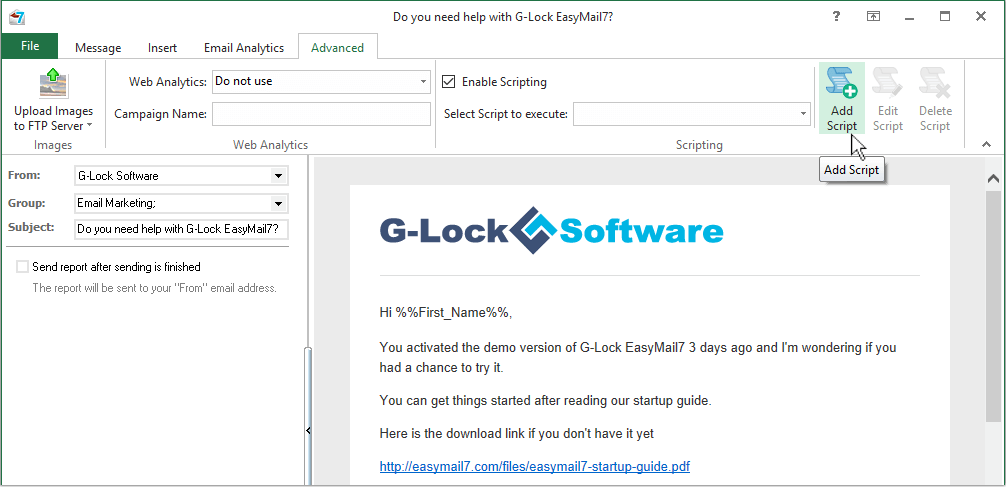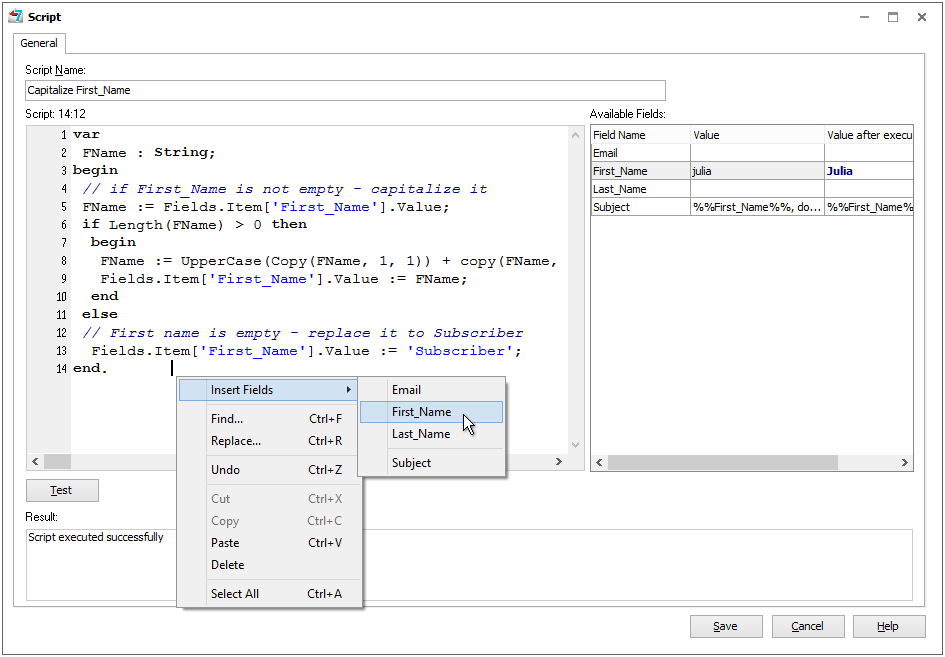# How to Execute a Script During Email Sending

In G-Lock EasyMail7 you can use the script to perform various operations with the fields values during email sending, for example, you can change the first lower case letter in the subscriber's first name into the upper case letter, replace a missing subscriber's name with any word for example "Subscriber", add http:// at the beginning of URLs, generate random numbers and use them in the email etc. All the fields in the contact group are available in the script.

The script works as a Pascal-like language interpreter. The basic differences from the standard Pascal are:

– all variables stored as variants;
– no need to declare variables.

## To add the script:

1. Open the message.

2. Select the account, group and type the Subject.

3. Click on the “Advanced” tab and check the "Enable Scripting" option.

4. Click on "Add Script".5. Enter a script name.

6. Type the script.

To insert field names into the script, click the right mouse button, click "Insert Fields" and select the field name from the menu.

7. To test if the script works as expected, type the values for the fields and click "Test".8. Click "Save" to save the script.

To edit the script, select the script and click "Edit Script".

To delete the script, click "Delete".

If you delete a script, it is deleted forever and won't be available for future messages. When you re-send a message with a script that was deleted, the message will be sent without the script execution.

## Here are a couple of sample scripts you can use:

### Script sample 1. The script below changes the first lower case letter in the subscriber's first name to the upper case letter and replaces a missing first name with the "Subscribe"word.

var
FName : String;
begin
// if First_Name is not empty – capitalize it
FName := Fields.Item[‘First_Name’].Value;
if Length(FName) > 0 then
begin
FName := UpperCase(Copy(FName, 1, 1)) + copy(FName, 2, 1024);
Fields.Item[‘First_Name’].Value := FName;
end
else
// First name is empty – replace it to Subscriber
Fields.Item[‘First_Name’].Value := 'Subscriber';
end.

### Script sample 2. The script below generates a random value containing a lower case letter, a number and an upper case letter and adds the random value to the Subject. (You can use any of the fields available in the group instead of the Subject field).

var
temps : string;
i : integer;
begin
Result := '';
temps := '';
Randomize;
if length(mask)=0  then exit;
if pos('datetime:',LowerCase(mask)) = 1
then
begin
result := formatdatetime(temps,Now);
exit;
end;

i := 1;
while i<= Length(mask) do
begin
if mask[i] = '\'
then
begin
inc(i);
then
temps := temps +mask[i];
inc(i);
continue;
end;
if mask[i] = 'd'
then
temps := temps + inttostr(Random(10))
else
if mask[i] = 'W'
then
temps := temps +chr(RandomRange(65,90))
else
if mask[i] = 'w'
then
temps := temps+chr(RandomRange(97,122))
else
inc(i);
end;
result := temps;
end;

begin
Fields.Item[‘Subject’].Value := Fields.Item[‘Subject’].Value + ' '+ GenerateValueByMask('wdW');
end.

### Script sample 3. This script replaces a dot in the value with a colon (2.5 -> 2,5).

Begin
Fields.Item[‘Purchase_price’].Value:= StringReplace(Fields.Item[‘Purchase_price’].Value, ‘.’,’,’,[rfReplaceAll]);
End.